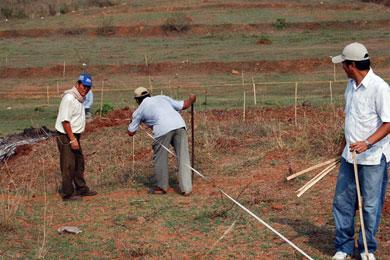# Measurement System in Nepal

35781In Nepal, where a Bigha is about 6,772.63 square meters. Officially, most measurement of lands use units of either Bigha (in Terai region) or Ropani (Nepali: रोपनी) (in Hilly regions). Metric system is very seldom used officially in measuring area of Land.

Measurement of area in terms of Bigha

1 bigha (बिघा)   = 20 kattha (कठ्ठा)   =   6,772.63 m²   = 72,900 sq.ft    = 13.31 ropani (रोपनी)
1 kattha (कठ्ठा)  = 20 dhur (धुर)        =  338.63 m²       = 3,645 sq.ft.
1 dhur (धुर)                                      =  16.93 m²         = 182.25 sq.ft

Measurement of area in terms of Ropani

1 ropani(रोपनी)   = 16 aana (आना)     =  508.72 m²       = 5476 sq. ft

1 aana(आना)       = 4 paisa (पैसा)       =  31.80 m²         = 342.25 sq.ft

1 paisa(पैसा)        = 4 daam (दाम)       =  7.95 m²          = 85.56 sq.ft
1daam (दाम)                                       =  1.99 m²          = 21.39 sq.ft
Also,
1 ropani(रोपनी)     = 4 matomuri
25 ropani(रोपनी)   = 1 khetmuri

Length Measurement

1 bitta       = 18 angul
1 haat       = 2 bitta
1 danda    = 4 haat
1 janjir      = 9 haat
1 kosh      = 480 danda      = 3.218 km    = 2 mile
1 gaj         = 0.9144 m       = 1 yard

Volume Measurement

1 moori         = 20 pathi
1 pathi          = 4 curuwa           = 4.54596 ltr
1 curuwa      = 2 mana
1 mana         = 4 chauthai      = 10 muthi
1 lit              = 1.7598 mana
Weight Measurement
1 kg              = 5 pau in ktm 4 pau outside ktm
1 pau            = 19 tola         = 199 gms
1 tola            = 100 lal         = 11.66 gm
1 chatak       = 4 1/2 tola     = 58.31 gm
1 pau           = 4 chatak
1 seer          = 0.9331 kg
1 dharni        = 12 pau          = 2.3934 kg
1 maund       = 37.3242 kg
1 gm             = 8.573 lal
1 pound       = 0.4536 kg